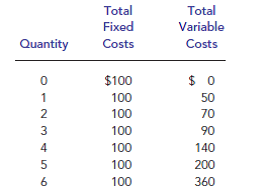Problem

# Ball Bearings Inc. faces costs of production as follows: a. Calculate the com...

Ball Bearings Inc. faces costs of production as follows:a. Calculate the company’s average fixed costs, average variable costs, average total costs, and marginal costs.

b. The price of a case of ball bearings is $50. Seeing that she can’t make a profit, the Chief Executive Officer (CEO) decides to shut down operations. What are the firm’s profits/ losses? Was this a wise decision? Explain. c. Vaguely remembering his introductory economics course, the Chief Financial Officer tells the CEO it is better to produce 1 case of ball bearings, because marginal revenue equals marginal cost at that quantity. What are the firm’s profits/losses at that level of production? Was this the best decision? Explain. #### Step-by-Step Solution Solution 1 771-14-5P SA: 3975  Q TFC TVC AFC AVC TC ATC MC TR MR Profit 0$100 $0 - -$100 - - $0 - ($100) 1 100 50 $100 50 150 150.00 50 50 50 ($100) 2 100 70 50 35 170 85.00 20 100 50 ($70) 3 100 90 33 30 190 63.33 20 150 50 ($40) 4 100 140 25 35 240 60.00 50 200 50 ($40) 5 100 200 20 40 300 60.00 60 250 50 ($50) 6 100 360 17 60 460 76.67 160 300 50 ($160) Q= Quantity TFC= total fixed cost TVC= total variable cost AFC= average fixed cost AVC= average variable cost TC= total cost ATC= Average total cost MC= marginal cost TR= total revenue MR= marginal revenue Profit: at all the production levels from 0 to 6 units loss is incurred. a) AFC= average fixed cost AFC= Total Fixed cost / output AFC at unit 1= 100/1 =$100

AFC at unit 2= 100/2

= $50 AFC at unit 3= 100/3 =$ 33.33

AVC= average variable cost

AVC= Total Variable cost/ output

AVC at unit 1= 50/1

= $50 TC= total cost Total cost is calculated by adding TFC and TVC TC= TFC + TVC TC for 2nd unit = 100 +70 ATC= Average total cost It is the average of Total cost, calculated by dividing TC with output ATC= TC/ Q ATC of 4th unit= TC at 4th unit/ 4 = 240/4 = 60 MC= marginal cost MC is the change in total cost for an additional unit of output. MC= TCn – TCn-1 MC for 3rd unit= TC3rd – TC2nd = 190 – 170 = 20 b) At Price of$50 per case, to know the profit or loss we have to calculate total revenue.

Profit= TR – TC

If TC exceeds TR than we can infer that it’s a loss.

TR, is the total revenue earned by the firm, it is calculated by multiplying output with price.

TR= Quantity x Price.

Profit (or loss) = TR – TC

Profit (or loss) at 4th unit is = TR – TC

= 200 – 240

= - 240

Negative revenue means a loss to the firm, we can observe from the above table that at every level of output the firm incurred a loss.

At every level of output the firm is incurring losses.

Options available:

When shut down: The firm incurs a loss of $100, which is the fixed cost component. By operating the firm when MR= MC at 4 units of output will cover all the operational expenses and also some part of the fixed cost component. So, it is advisable to operate the firm and produce an output of 4 units, where the loss is minimized to$40, otherwise firm has to bear a loss of $100. c) Profit maximization output is produced when MR=MC, but at 1 unit output the firm incurs a loss of$100.

Instead it can go for 4 units of output, MR=MC= 50, and at this level of output all the variable expenses are covered and some part of fixed expenses are also covered by minimize the loss to \$40.